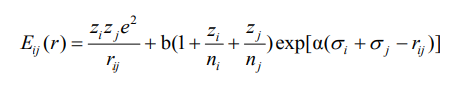# Problems in the use of the BMH potential function

Why is the BMH potential function expressed in the literature in this formwhile in the lammps handbook it does look like thisIf I want to use the first form, how do I write the pair-style command？
Any help would be greatly appreciated.

It is not just the LAMMPS manual. It is elsewhere, too (e.g. Born-Huggins-Meyer potential page on SklogWiki - a wiki for statistical mechanics and thermodynamics), and I suspect that the literature you are quoting is the outlier and it is - as far as I can tell - a different potential.

Presumably you can run this in LAMMPS by writing each pair coeff as:

\rho = 1/\alpha

A = b(1+z_i/n_i + z_j/n_j)

\sigma = \sigma_i + \sigma_j

C = D = 0